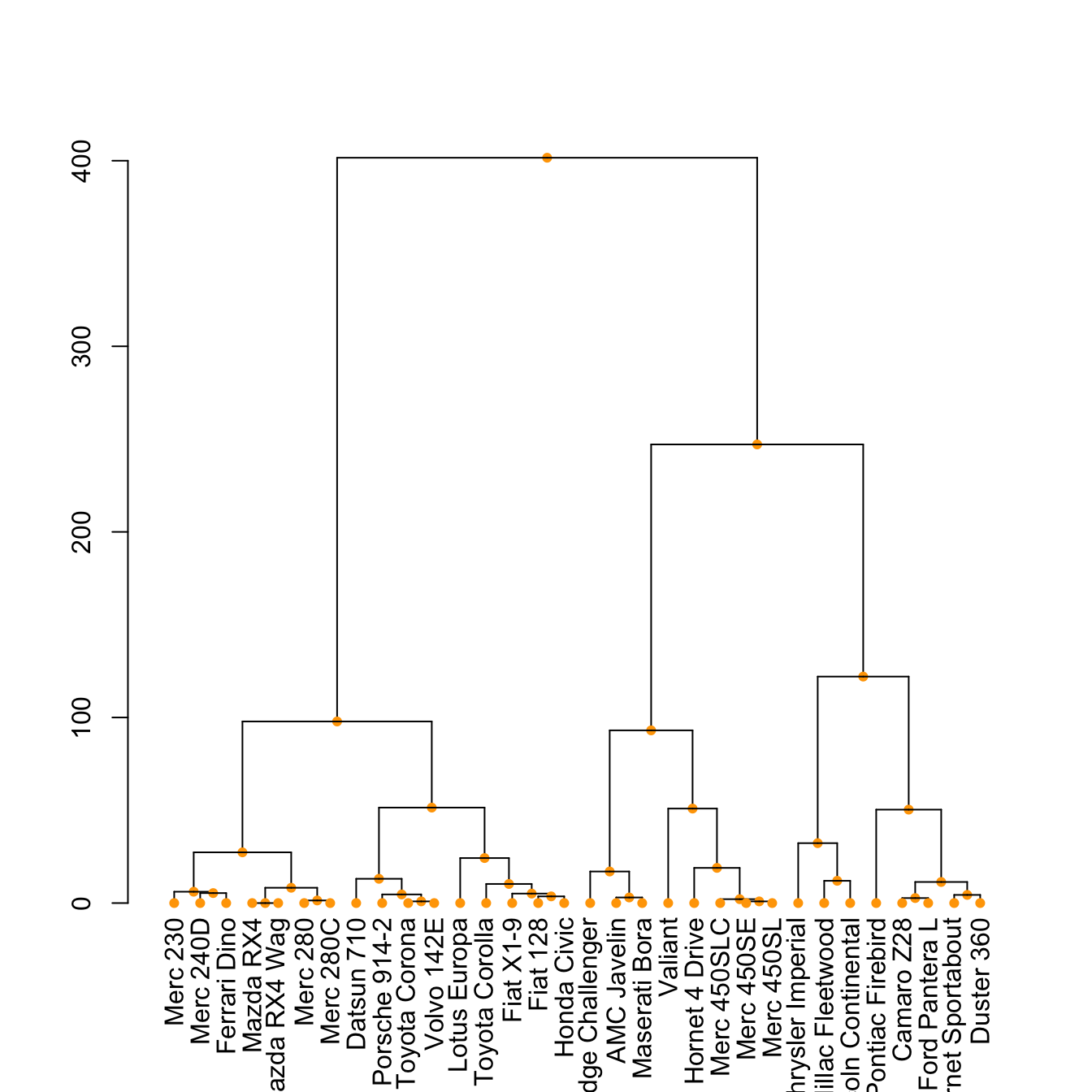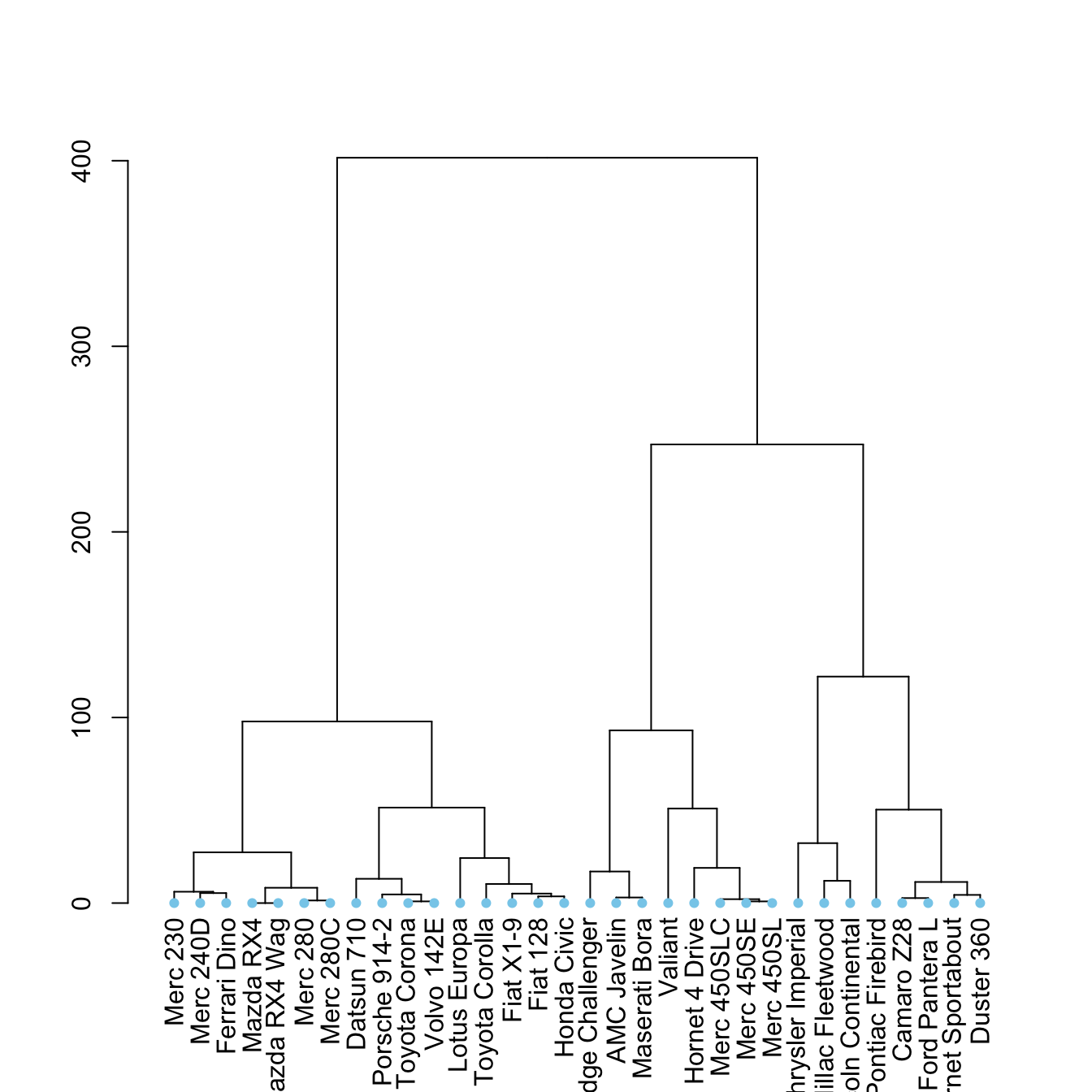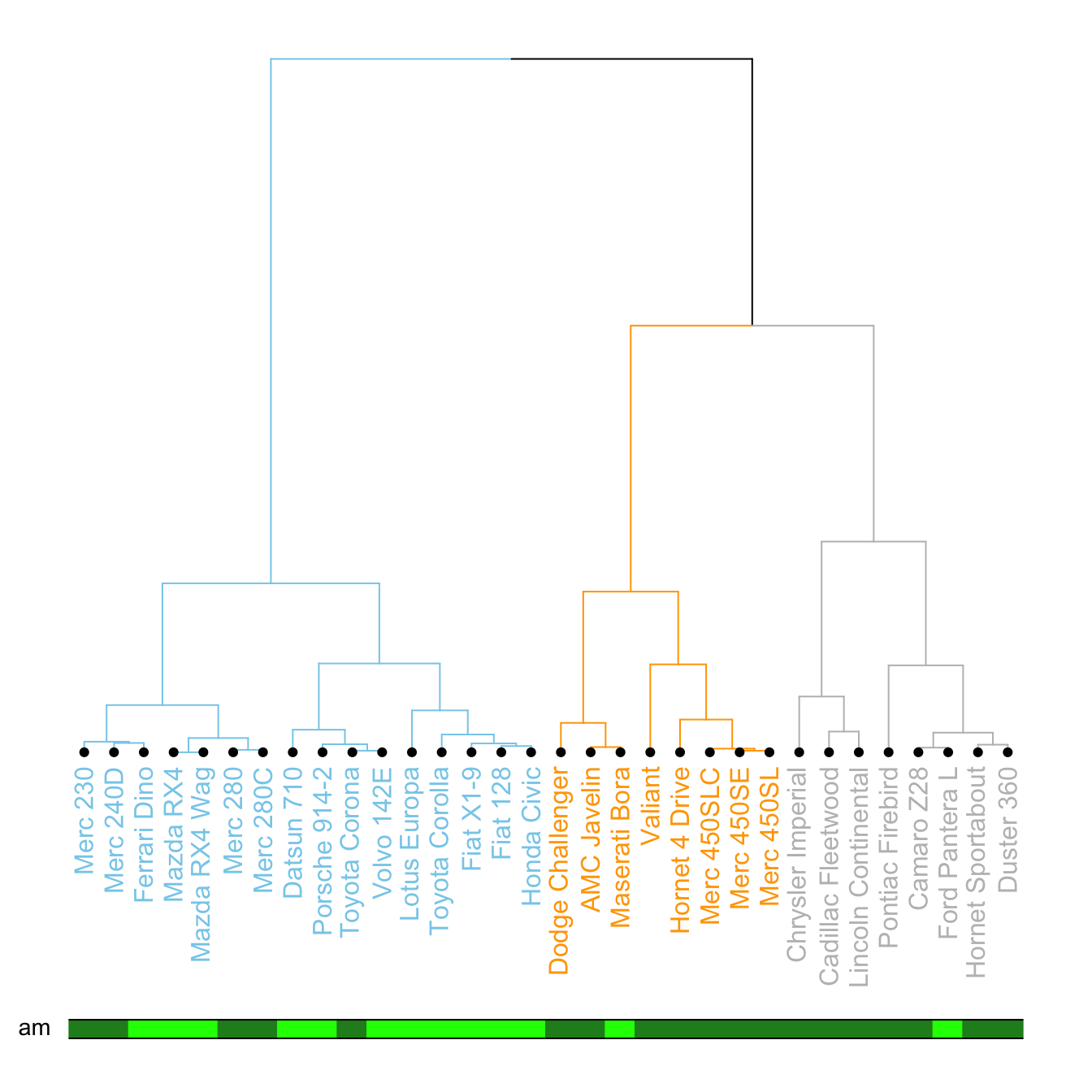# Customized dendrogram with R and the dendextend package

The `dendextend` package allows to apply all kinds of customization to a dendrogram: coloring nodes, labels, putting several tree face to face and more.

# Basic dendrogram

First of all, let’s remind how to build a basic dendrogram with `R`:

• input dataset is a dataframe with individuals in row, and features in column
• `dist()` is used to compute distance between sample
• `hclust()` performs the hierarchical clustering
• the `plot()` function can plot the output directly as a tree``````# Library
library(tidyverse)

# Data

# Clusterisation using 3 variables
mtcars %>%
select(mpg, cyl, disp) %>%
dist() %>%
hclust() %>%
as.dendrogram() -> dend

# Plot
par(mar=c(7,3,1,1))  # Increase bottom margin to have the complete label
plot(dend)``````

# The `set()` functionThe `set()` function of `dendextend` allows to modify the attribute of a specific part of the tree.

You can customize the `cex`, `lwd`, `col`, `lty` for `branches` and `labels` for example. You can also custom the nodes or the leaf. The code below illustrates this concept:

``````# library
library(dendextend)

# Chart (left)
dend %>%
# Custom branches
set("branches_col", "grey") %>% set("branches_lwd", 3) %>%
# Custom labels
set("labels_col", "orange") %>% set("labels_cex", 0.8) %>%
plot()``````
``````# Middle
dend %>%
set("nodes_pch", 19)  %>%
set("nodes_cex", 0.7) %>%
set("nodes_col", "orange") %>%
plot()``````
``````# right
dend %>%
set("leaves_pch", 19)  %>%
set("leaves_cex", 0.7) %>%
set("leaves_col", "skyblue") %>%
plot()``````

# Highlight clustersThe `dendextend` library has some good functionalities to highlight the tree clusters.

You can color branches and label following their cluster attribution, specifying the number of cluster you want. The `rect.dendrogram()` function even allows to highlight one or several specific clusters with a rectangle.

``````# Color in function of the cluster
par(mar=c(1,1,1,7))
dend %>%
set("labels_col", value = c("skyblue", "orange", "grey"), k=3) %>%
set("branches_k_color", value = c("skyblue", "orange", "grey"), k = 3) %>%
plot(horiz=TRUE, axes=FALSE)
abline(v = 350, lty = 2)``````
``````# Highlight a cluster with rectangle
par(mar=c(9,1,1,1))
dend %>%
set("labels_col", value = c("skyblue", "orange", "grey"), k=3) %>%
set("branches_k_color", value = c("skyblue", "orange", "grey"), k = 3) %>%
plot(axes=FALSE)
rect.dendrogram( dend, k=3, lty = 5, lwd = 0, x=1, col=rgb(0.1, 0.2, 0.4, 0.1) ) ``````

# Comparing with an expected clustering

It is a common task to compare the cluster you get with an expected distribution.

In the `mtcars` dataset we used to build our dendrogram, there is an `am` column that is a binary variable. We can check if this variable is consistent with the cluster we got using the `colored_bars()` function.``````# Create a vector of colors, darkgreen if am is 0, green if 1.
my_colors <- ifelse(mtcars\$am==0, "forestgreen", "green")

# Make the dendrogram
par(mar=c(10,1,1,1))
dend %>%
set("labels_col", value = c("skyblue", "orange", "grey"), k=3) %>%
set("branches_k_color", value = c("skyblue", "orange", "grey"), k = 3) %>%
set("leaves_pch", 19)  %>%
set("nodes_cex", 0.7) %>%
plot(axes=FALSE)

colored_bars(colors = my_colors, dend = dend, rowLabels = "am")``````

# Comparing 2 dendrograms with `tanglegram()`

It is possible to compare 2 dendrograms using the `tanglegram()` function.

Here it illustrates a very important concept: when you calculate your distance matrix and when you run your hierarchical clustering algorithm, you cannot simply use the default options without thinking about what you’re doing. Have a look to the differences between 2 different methods of clusterisation.``````# Make 2 dendrograms, using 2 different clustering methods
d1 <- USArrests %>% dist() %>% hclust( method="average" ) %>% as.dendrogram()
d2 <- USArrests %>% dist() %>% hclust( method="complete" ) %>% as.dendrogram()

# Custom these kendo, and place them in a list
dl <- dendlist(
d1 %>%
set("labels_col", value = c("skyblue", "orange", "grey"), k=3) %>%
set("branches_lty", 1) %>%
set("branches_k_color", value = c("skyblue", "orange", "grey"), k = 3),
d2 %>%
set("labels_col", value = c("skyblue", "orange", "grey"), k=3) %>%
set("branches_lty", 1) %>%
set("branches_k_color", value = c("skyblue", "orange", "grey"), k = 3)
)

# Plot them together
tanglegram(dl,
common_subtrees_color_lines = FALSE, highlight_distinct_edges  = TRUE, highlight_branches_lwd=FALSE,
margin_inner=7,
lwd=2
)``````

Related chart types

## Contact

This document is a work by Yan Holtz. Any feedback is highly encouraged. You can fill an issue on Github, drop me a message on Twitter, or send an email pasting yan.holtz.data with gmail.com.